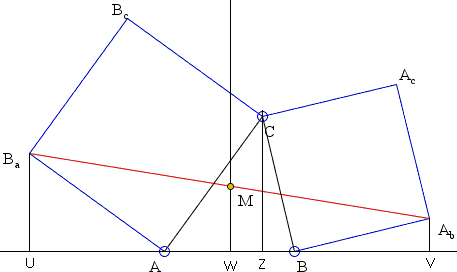# An Elementary Proof of Bottema's Theorem

Consider a configuration of two squares ACBcBa and BCAcAb with a common vertex C.Bottema's theorem claims that the midpoint M of the segment AbBa is independent of C.

### Proof

Let's drop perpendiculars BaU, MW, AbV, and CZ onto AB.MW is the midline of trapezoid BaUVAb so that

MW = (BaU + AbV) / 2.

Further, since ∠BaAC is right, angles BaAU and CAZ are complementary which makes right triangles BaAU and ACZ equal, implying

BaU = AZ.

Similarly,

AbV = BZ.

Taking all three identities into account shows that

MW = (BaU + AbV) / 2 = (AZ + BZ) / 2 = AB/2 = AW,

independent of C. (The proof also shows that M is the center of the square formed on AB upwards.)

Note: there is a dynamic illustration of the proof that makes it entirely obvious.

### Reference

1. A. Shriki, Back To Treasure Island, MathematicS Teacher, Vol. 104, No. 9 (May 2011), 658-664### Bottema's TheoremCopyright © 1996-2018 Alexander Bogomolny

 65271689